Published: 30 June 2013

# Analytical periodic solution for solving nonlinear vibration equations

M. Bayat1
I. Pakar2
M. Bayat3
1Young Researchers and Elites Club, Science and Research Branch, Islamic Azad University, Tehran, Iran
Corresponding Author:
M. Bayat
Views 27

#### Abstract

In this study a new kind of analytical methods called He’s variational approach method is applied to solve strong nonlinear vibration equations. The He’s variational approach method is very easy and contrary to the other conventional methods, only one iteration leads to high accuracy of the solutions for the whole range of initial amplitudes and does not demand small perturbation. Some examples are given to illustrate the effectiveness and convenience of the methodology. The Runge-Kutta’s (RK) algorithm was also implemented to achieve the numerical solutions for the examples. The results reveal that the variational approach method is very effective and simple. It is predicted that the VAM can find wide application in engineering problems, as indicated in following examples.

## 1. Introduction

Finding an analytical solution for nonlinear vibration problems has been a topic of intensive research for many years.

In the past recent decades some powerful and new analytical methods have been considered by many researchers, such as: homotopy perturbation method , energy balance method [2-5], amplitude frequency formulation , iteration perturbation method , max-min approach [8-10], variational approach method [11-16], homotopy analysis method , and the other analytical and numerical methods [18-24].

Among these methods, Variational Approach Method (VAM) is considered to solve the nonlinear vibration equations in this paper.

The paper has been collocated as follows.

The basic idea of He’s variational approach is introduced in section 2. In section 3 for numerical solution of nonlinear equation the algorithm of Runge-Kutta method is described. Then in section 4 applications of He’s variational approach method have been studied, to demonstrate the applicability and preciseness of the method. In section 5 some comparisons between analytical and numerical solutions are presented. Eventually we show that VAM can converge to a precise cyclic solution for nonlinear systems.

## 2. Basic concept of variational approach method

He suggested a variational approach which is different from the known variational methods in open literature . Hereby we give a brief introduction of the method:

1
$\stackrel{¨}{u}+f\left(u\right)=0.$

Its variational principle can be easily established utilizing the semi-inverse method:

2
$J\left(u\right)={\int }_{0}^{T/4}\left(-\frac{1}{2}{\stackrel{˙}{u}}^{2}+F\left(u\right)\right)dt,$

where $T$ is period of the nonlinear oscillator, $\partial F}{\partial u}=f$. Assume that its solution can be expressed as:

3
$u\left(t\right)=A\mathrm{c}\mathrm{o}\mathrm{s}\left(\omega t\right),$

where $A$ and $\omega$ are the amplitude and frequency of the oscillator, respectively. Substituting Eq. (3) into Eq. (2) results in:

4
$J\left(A,\omega \right)={\int }_{0}^{\frac{T}{4}}\left(-\frac{1}{2}{A}^{2}{\omega }^{2}{\mathrm{s}\mathrm{i}\mathrm{n}}^{2}\omega t+F\left(A\mathrm{c}\mathrm{o}\mathrm{s}\omega t\right)\right)dt=$
$\frac{1}{\omega }{\int }_{0}^{\frac{\pi }{2}}\left(-\frac{1}{2}{A}^{2}{\omega }^{2}{\mathrm{s}\mathrm{i}\mathrm{n}}^{2}t+F\left(A\mathrm{c}\mathrm{o}\mathrm{s}t\right)\right)dt=-\frac{1}{2}{A}^{2}\omega {\int }_{0}^{\frac{\pi }{2}}{\mathrm{s}\mathrm{i}\mathrm{n}}^{2}t\text{\hspace{0.17em}}dt+\frac{1}{\omega }{\int }_{0}^{\frac{\pi }{2}}F\left(A\mathrm{c}\mathrm{o}\mathrm{s}t\right)dt.$

Applying the Ritz method, He requires:

5
$\frac{\partial J}{\partial A}=0,$
6
$\frac{\partial J}{\partial \omega }=0.$

But with a careful inspection, for most cases He found that:

7
$\frac{\partial J}{\partial \omega }=-\frac{1}{2}{A}^{2}{\int }_{0}^{\frac{\pi }{2}}{\mathrm{s}\mathrm{i}\mathrm{n}}^{2}t\text{\hspace{0.17em}}dt-\frac{1}{{\omega }^{2}}{\int }_{0}^{\frac{\pi }{2}}F\left(A\mathrm{c}\mathrm{o}\mathrm{s}t\right)\text{\hspace{0.17em}\hspace{0.17em}}dt<0.$

Thus He modified conditions Eq. (5) and Eq. (6) into a simpler form:

8
$\frac{\partial J}{\partial \omega }=0,$

from which the relationship between the amplitude and frequency of the oscillator can be obtained.

## 3. Basic concept of Runge-Kutta method

For the numerical approach to verify the analytic solution, the fourth order RK (Runge-Kutta) method has been used. This iterative algorithm is written in the form of the following formulae for the second-order differential equation:

9
${\stackrel{˙}{u}}_{i+1}={\stackrel{˙}{u}}_{i}+\frac{\Delta t}{6}\left({h}_{1}+2{h}_{2}+2{h}_{3}+{k}_{4}\right),$
${u}_{i+1}={u}_{i}+\Delta t\left({\stackrel{˙}{u}}_{i}+\frac{\Delta t}{6}\left({h}_{1}+{h}_{2}+{k}_{3}\right)\right),$

where $\Delta t$ is the increment of the time and ${h}_{1}$, ${h}_{2}$, ${h}_{3}$ and ${h}_{4}$ are determined from the following formulae:

10
${h}_{1}=f\left(\stackrel{˙}{u},{u}_{i},{\stackrel{˙}{u}}_{i}\right)k,$
${h}_{2}=f\left({t}_{i}+\frac{\Delta t}{2},\text{\hspace{0.17em}\hspace{0.17em}\hspace{0.17em}\hspace{0.17em}}{u}_{i}+\frac{\Delta t}{2}{\stackrel{˙}{u}}_{i},\text{\hspace{0.17em}\hspace{0.17em}\hspace{0.17em}}{\stackrel{˙}{u}}_{i}+\frac{\Delta t}{2}{h}_{1}\right),$
${h}_{3}=f\left({t}_{i}+\frac{\Delta t}{2},\text{\hspace{0.17em}\hspace{0.17em}\hspace{0.17em}}{u}_{i}+\frac{\Delta t}{2}{\stackrel{˙}{u}}_{i},\text{\hspace{0.17em}\hspace{0.17em}\hspace{0.17em}\hspace{0.17em}\hspace{0.17em}}\frac{1}{4}\Delta {t}^{2}{h}_{1},\text{\hspace{0.17em}\hspace{0.17em}\hspace{0.17em}\hspace{0.17em}}{\stackrel{˙}{u}}_{i}+\frac{\Delta t}{2}{h}_{2}\right),$
${h}_{4}=f\left({t}_{i}+\Delta t,\text{\hspace{0.17em}\hspace{0.17em}\hspace{0.17em}\hspace{0.17em}}{u}_{i}+\Delta t\text{\hspace{0.17em}}{\stackrel{˙}{u}}_{i},\text{\hspace{0.17em}\hspace{0.17em}\hspace{0.17em}}\frac{1}{2}\Delta {t}^{2}{h}_{2},\text{\hspace{0.17em}\hspace{0.17em}\hspace{0.17em}\hspace{0.17em}}{\stackrel{˙}{u}}_{i}+\Delta t\text{\hspace{0.17em}}{h}_{3}\right).$

The numerical solution starts from the boundary at the initial time, where the first value of the displacement function and its first-order derivative are determined from initial condition. Then with a small time increment $\Delta t$ the displacement function and its first-order derivative at the new position can be obtained using Eq. (10). This process continues to the end of the time limit.

## 4. Application

In order to show the accuracy of the new approach method, we will apply the method for the following two examples.

### 4.1. Example 1

We consider the physical model of nonlinear equation in the following figure with $F\left(t\right)={F}_{0}\mathrm{s}\mathrm{i}\mathrm{n}{\omega }_{0}t$ indicated in Figure 1.

The motion equation is:

11
$\stackrel{¨}{\theta }+\frac{4k}{3m}\mathrm{s}\mathrm{i}\mathrm{n}\theta -\frac{3{F}_{0}}{ml}\mathrm{s}\mathrm{i}\mathrm{n}{\omega }_{0}t=0,\text{\hspace{0.17em}\hspace{0.17em}\hspace{0.17em}\hspace{0.17em}\hspace{0.17em}\hspace{0.17em}\hspace{0.17em}\hspace{0.17em}\hspace{0.17em}\hspace{0.17em}\hspace{0.17em}\hspace{0.17em}}\theta \left(0\right)=A,\stackrel{˙}{\theta }\left(0\right)=0.$

Fig. 1The physical model of nonlinear equation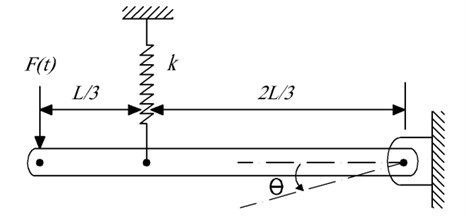This equation is known as Mathieu equation or the system with dependent coefficients to time, in which $\theta$ and $t$ are generalized dimensionless displacements and time variables, respectively.

The approximation $\mathrm{s}\mathrm{i}\mathrm{n}\left(\theta \right)=\theta -\left(1/6\right){\theta }^{3}+\left(1/120\right){\theta }^{5}$ is used.

Its variational formulation can be readily obtained from Eq. (11) as follows:

12
$J\left(\theta \right)=\text{\hspace{0.17em}}{\int }_{0}^{t}\left(\frac{1}{2}{\stackrel{¨}{\theta }}^{2}+\frac{2}{3}\frac{k}{m}{\theta }^{2}-\frac{1}{18}\frac{k}{m}{\theta }^{4}+\frac{1}{540}\frac{k}{m}{\theta }^{6}-\frac{3{F}_{0}\mathrm{sin}\left({\omega }_{0}t\right)}{ml}\theta \right)dt.$

Choosing the trial function $\theta \left(t\right)=A\mathrm{c}\mathrm{o}\mathrm{s}\text{\hspace{0.17em}}\left(\omega t\right)$ into Eq. (12) we obtain:

13
$J\left(A\right)={\int }_{0}^{T/4}\left(\begin{array}{c}\frac{1}{2}{A}^{2}{\omega }^{2}{\mathrm{s}\mathrm{i}\mathrm{n}}^{2}\left(\omega \text{\hspace{0.17em}}t\right)+\frac{2}{3}\frac{k}{m}{A}^{2}{\mathrm{c}\mathrm{o}\mathrm{s}}^{2}\left(\omega \text{\hspace{0.17em}}t\right)-\frac{1}{18}\frac{k}{m}{A}^{4}{\mathrm{c}\mathrm{o}\mathrm{s}}^{4}\left(\omega \text{\hspace{0.17em}}t\right)\\ +\frac{1}{540}\frac{k}{m}{A}^{6}{\mathrm{c}\mathrm{o}\mathrm{s}}^{6}\left(\omega \text{\hspace{0.17em}}t\right)-\frac{3{F}_{0}\mathrm{sin}\left({\omega }_{0}t\right)}{ml}Acos\left(\omega \text{\hspace{0.17em}}t\right)\end{array}\right)dt\text{\hspace{0.17em}}.$

The stationary condition with respect to $A$ leads to:

14
$\frac{\partial J}{\partial A}={\int }_{0}^{T/4}\left(\begin{array}{c}A{\omega }^{2}{\mathrm{s}\mathrm{i}\mathrm{n}}^{2}\left(\omega \text{\hspace{0.17em}}t\right)+\frac{4}{3}\frac{k}{m}A{\mathrm{c}\mathrm{o}\mathrm{s}}^{2}\left(\omega \text{\hspace{0.17em}}t\right)-\frac{2}{9}\frac{k}{m}{A}^{3}{\mathrm{c}\mathrm{o}\mathrm{s}}^{4}\left(\omega \text{\hspace{0.17em}}t\right)\\ +\frac{1}{90}\frac{k}{m}{A}^{5}{\mathrm{c}\mathrm{o}\mathrm{s}}^{6}\left(\omega \text{\hspace{0.17em}}t\right)-\frac{3{F}_{0}\mathrm{sin}\left({\omega }_{0}t\right)}{ml}\mathrm{cos}\left(\omega \text{\hspace{0.17em}}t\right)\end{array}\right)\text{\hspace{0.17em}}dt=0,$

or:

15
$\frac{\partial J}{\partial A}={\int }_{0}^{\pi /2}\left(\begin{array}{c}A{\omega }^{2}{\mathrm{s}\mathrm{i}\mathrm{n}}^{2}t+\frac{4}{3}\frac{k}{m}A{\mathrm{c}\mathrm{o}\mathrm{s}}^{2}t-\frac{2}{9}\frac{k}{m}{A}^{3}{\mathrm{c}\mathrm{o}\mathrm{s}}^{4}t\\ +\frac{1}{90}\frac{k}{m}{A}^{5}{\mathrm{c}\mathrm{o}\mathrm{s}}^{6}t-\frac{3{F}_{0}\mathrm{sin}\left({\omega }_{0}t\right)}{ml}cost\end{array}\right)\text{\hspace{0.17em}}dt=0.$

Solving Eq. (15) according to $\omega$ we have:

16
$\omega {\text{\hspace{0.17em}}}^{2}=\frac{{\int }_{0}^{\frac{\pi }{2}}\left(\frac{4}{3}\frac{k}{m}A{\mathrm{c}\mathrm{o}\mathrm{s}}^{2}t-\frac{2}{9}\frac{k}{m}{A}^{3}{\mathrm{c}\mathrm{o}\mathrm{s}}^{4}t+\frac{1}{90}\frac{k}{m}{A}^{5}{\mathrm{c}\mathrm{o}\mathrm{s}}^{6}t-\frac{3{F}_{0}\mathrm{sin}\left({\omega }_{0}t\right)}{ml}\mathrm{c}\mathrm{o}\mathrm{s}t\right)dt}{{\int }_{0}^{\frac{\pi }{2}}A\text{\hspace{0.17em}}{\mathrm{s}\mathrm{i}\mathrm{n}}^{2}t\text{\hspace{0.17em}}dt}.$

Then we have:

17
$\omega {\text{\hspace{0.17em}}}_{VAM}=\frac{1}{12}\sqrt{\frac{1728{F}_{0}\mathrm{sin}\left(\frac{1}{2}\pi {\omega }_{0}\right)-1728{F}_{0}{\omega }_{0}+kAl\pi \left({\omega }_{0}^{2}-1\right)\left(192+{A}^{4}-24{A}^{2}\right)}{\left(m{\omega }_{0}^{2}-m\right)lA\pi }}.$

According to Eqs. (3) and (17) we can obtain the following approximate solution:

18
$\theta \left(t\right)=A\text{\hspace{0.17em}}\mathrm{cos}\left(\frac{1}{12}\sqrt{\frac{1728{F}_{0}\mathrm{sin}\left(\frac{1}{2}\pi {\omega }_{0}\right)-1728{F}_{0}{\omega }_{0}+kAl\pi \left({{\omega }_{0}}^{2}-1\right)\left(192+{A}^{4}-24{A}^{2}\right)}{\left(m{{\omega }_{0}}^{2}-m\right)lA\pi }}\text{\hspace{0.17em}}t\right).$

In Figures 3 and 4 we compared variational approach method with numerical solution for different parameters.

### 4.2. Example 2

We consider the motion equation of the pendulum with harmonic stringer point in Figure 2.

The solution of Mathieu equation of pendulum with harmonic stringer point according to the variational approach method is:

19
$\stackrel{¨}{\theta }+\left(\frac{g}{l}-\frac{{\omega }_{0}^{2}Y}{l}\mathrm{c}\mathrm{o}\mathrm{s}{\omega }_{0}t\right)\mathrm{s}\mathrm{i}\mathrm{n}\theta =0,\mathrm{}\mathrm{}\theta \left(0\right)=A,\stackrel{˙}{\theta }\left(0\right)=0.$

This equation is known as Mathieu equation or the system with dependent coefficients to time, in which $\theta$ and $t$ are generalized dimensionless displacements and time variables, respectively.

The approximation $\mathrm{s}\mathrm{i}\mathrm{n}\left(\theta \right)=\theta -\left(1/6\right){\theta }^{3}$ is used.

Its variational formulation can be readily obtained from Eq. (19) as follows:

20
$J\left(\theta \right)=\text{\hspace{0.17em}}{\int }_{0}^{t}\left(\frac{1}{2}{\stackrel{¨}{\theta }}^{2}+\frac{1}{2}\left(\frac{g}{l}-\frac{{\omega }_{0}^{2}\text{\hspace{0.17em}}Y\mathrm{c}\mathrm{o}\mathrm{s}\left({\omega }_{0}t\right)}{l}\right){\theta }^{2}+\frac{1}{24}\left(\frac{g}{l}-\frac{{\omega }_{0}^{2}\text{\hspace{0.17em}}Y\mathrm{c}\mathrm{o}\mathrm{s}\left({\omega }_{0}t\right)}{l}\right){\theta }^{4}\right)\text{\hspace{0.17em}}dt\text{\hspace{0.17em}}.$

Fig. 2Pendulum with harmonic stringer point: y(t)=Ycosω0 t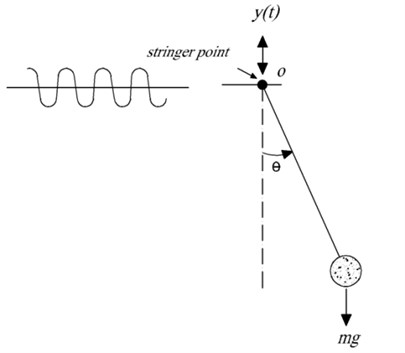Choosing the trial function $\theta \left(t\right)=A\mathrm{c}\mathrm{o}\mathrm{s}\left(\omega \text{\hspace{0.17em}}t\right)$ into Eq. (18) we obtain:

21
$J\left(A\right)={\int }_{0}^{T/4}\left(\begin{array}{c}\frac{1}{2}{A}^{2}{\omega }^{2}{\mathrm{s}\mathrm{i}\mathrm{n}}^{2}\left(\omega \text{\hspace{0.17em}}t\right)+\frac{1}{2}{A}^{2}\left(\frac{g}{l}-\frac{{{\omega }_{0}}^{2}Y\mathrm{c}\mathrm{o}\mathrm{s}\left({\omega }_{0}\text{\hspace{0.17em}}t\right)}{l}\right){\mathrm{c}\mathrm{o}\mathrm{s}}^{2}\left(\omega \text{\hspace{0.17em}}t\right)\\ +\frac{1}{24}{A}^{4}\left(\frac{g}{l}-\frac{{{\omega }_{0}}^{2}Y\mathrm{c}\mathrm{o}\mathrm{s}\left({\omega }_{0}\text{\hspace{0.17em}}t\right)}{l}\right){\mathrm{c}\mathrm{o}\mathrm{s}}^{4}\left(\omega \text{\hspace{0.17em}}t\right)\end{array}\right)\text{\hspace{0.17em}}dt.$

The stationary condition with respect to $A$ leads to:

22
$\frac{\partial J}{\partial A}={\int }_{0}^{T/4}\left(\begin{array}{c}A\text{\hspace{0.17em}}{\omega }^{2}\text{\hspace{0.17em}}{\mathrm{s}\mathrm{i}\mathrm{n}}^{2}\left(\omega t\right)+A\left(\frac{g}{l}-\frac{{\omega }_{0}^{2}\text{\hspace{0.17em}}Y\mathrm{c}\mathrm{o}\mathrm{s}\left({\omega }_{0}t\right)}{l}\right){\mathrm{c}\mathrm{o}\mathrm{s}}^{2}\left(\omega t\right)\\ +\frac{1}{6}A\left(\frac{g}{l}-\frac{{\omega }_{0}^{2}\text{\hspace{0.17em}}Y\mathrm{c}\mathrm{o}\mathrm{s}\left({\omega }_{0}t\right)}{l}\right){\mathrm{c}\mathrm{o}\mathrm{s}}^{4}\left(\omega t\right)\end{array}\right)\text{\hspace{0.17em}}dt=0,$

or:

23
$\frac{\partial J}{\partial A}={\int }_{0}^{\pi /2}\left(\begin{array}{c}A\text{\hspace{0.17em}}{\omega }^{2}\text{\hspace{0.17em}}{\mathrm{s}\mathrm{i}\mathrm{n}}^{2}\text{\hspace{0.17em}}t+A\left(\frac{g}{l}-\frac{{\omega }_{0}^{2}\text{\hspace{0.17em}}Y\mathrm{c}\mathrm{o}\mathrm{s}\left({\omega }_{0}t\right)}{l}\right){\mathrm{c}\mathrm{o}\mathrm{s}}^{2}\text{\hspace{0.17em}}t\\ +\frac{1}{6}A\left(\frac{g}{l}-\frac{{\omega }_{0}^{2}\text{\hspace{0.17em}}Y\mathrm{c}\mathrm{o}\mathrm{s}\left({\omega }_{0}t\right)}{l}\right){\mathrm{c}\mathrm{o}\mathrm{s}}^{4}\text{\hspace{0.17em}}t\end{array}\right)\text{\hspace{0.17em}}dt=0.$

Solving Eq. (23) according to $\omega$ we have:

24
$\omega {\text{\hspace{0.17em}}}^{2}=\frac{{\int }_{0}^{\frac{\pi }{2}}\left(\left(\frac{g}{l}-\frac{{\omega }_{0}^{2}\text{\hspace{0.17em}}Y\mathrm{c}\mathrm{o}\mathrm{s}\left({\omega }_{0}t\right)}{l}\right){\mathrm{c}\mathrm{o}\mathrm{s}}^{2}\text{\hspace{0.17em}}t+\frac{1}{6}A\left(\frac{g}{l}-\frac{{\omega }_{0}^{2}\text{\hspace{0.17em}}Y\mathrm{c}\mathrm{o}\mathrm{s}\left({\omega }_{0}t\right)}{l}\right){\mathrm{c}\mathrm{o}\mathrm{s}}^{4}\text{\hspace{0.17em}}t\right)\text{\hspace{0.17em}}dt}{{\int }_{0}^{\frac{\pi }{2}}A\text{\hspace{0.17em}\hspace{0.17em}}{\mathrm{s}\mathrm{i}\mathrm{n}}^{2}\text{\hspace{0.17em}}t\text{\hspace{0.17em}}dt}.$

Fig. 3Comparison of analytical solution of θ based on time with the numerical solution for cases: a) L=0.5 m, m=20 kg, k=800 N/m, F0=1 N, ω0=2 rad/sec, A=π/6, b) L=1 m, m=10 kg, k=1200 N/m, F0=1 N, ω0=2 rad/sec, A=π/3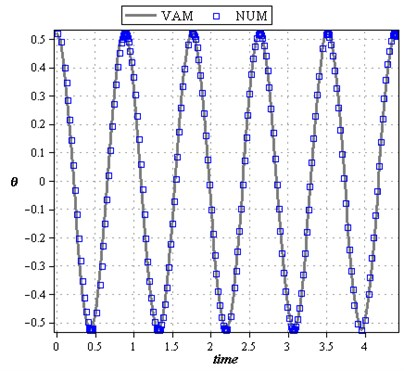a)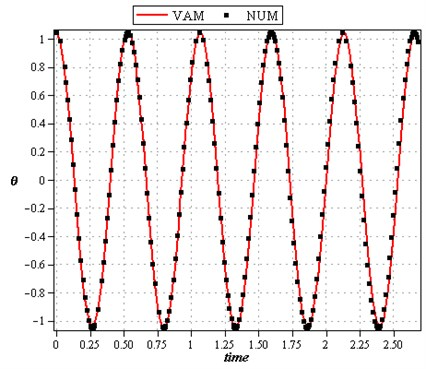b)

Fig. 4Comparison of phase plan of the analytical solution with the numerical solution for cases: a) L=0.5 m, m=20 kg, k=800 N/m, F0=1 N, ω0=2 rad/sec, A=π/6, b) L=1 m, m=10 kg, k=1200 N/m, F0=1 N, ω0=2 rad/sec, A=π/3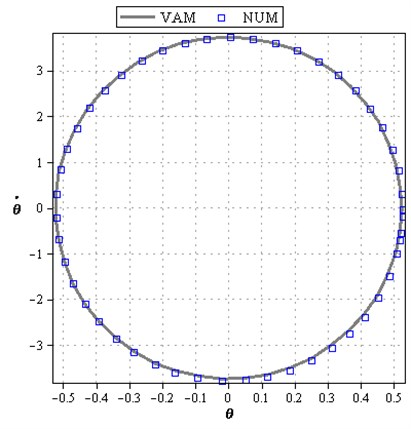a)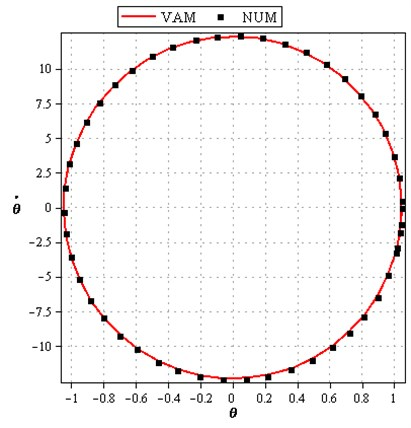b)

Then we have:

25
$\omega {\text{\hspace{0.17em}}}_{VAM}=\frac{\sqrt{2}}{4}\sqrt{\frac{128\text{\hspace{0.17em}}Y\left({A}^{2}+\frac{1}{2}{\omega }_{0}^{2}-8\right){\omega }_{0}\mathrm{sin}\left(\frac{1}{2}\pi {\omega }_{0}\right)-g\pi \left({\omega }_{0}^{2}-16\right)\left({\omega }_{0}^{2}-4\right)\left({A}^{2}-8\right)}{l\left({\omega }_{0}^{4}-20{\omega }_{0}^{2}+64\right)\text{\hspace{0.17em}}\pi }}.$

According to Eqs. (3) and (25) we can obtain the following approximate solution:

26
$\theta \left(t\right)=A\text{\hspace{0.17em}}\mathrm{c}\mathrm{o}\mathrm{s}\text{\hspace{0.17em}}\left(\frac{\sqrt{2}}{4}\sqrt{\frac{128\text{\hspace{0.17em}}Y\left({A}^{2}+\frac{1}{2}{\omega }_{0}^{2}-8\right){\omega }_{0}\mathrm{sin}\left(\frac{1}{2}\pi {\omega }_{0}\right)-g\pi \left({\omega }_{0}^{2}-16\right)\left({\omega }_{0}^{2}-4\right)\left({A}^{2}-8\right)}{l\left({\omega }_{0}^{4}-20{\omega }_{0}^{2}+64\right)\text{\hspace{0.17em}}\pi }}\text{\hspace{0.17em}}t\right).$

For this example we consider different parameters to show the accuracy of the method.

Figures 5 and 6 show the excellent agreement of the variational approach method with the numerical one.

Fig. 5Comparison of analytical solution of θ t based on time with the numerical solution: a) L=0.5 m, ω0=1rad/sec, Y=0.25 m, g=9.81m/s2, A=π/6, b) L=1 m, ω0=1 rad/sec, Y=0.5 m, g=9.81 m/s2, A=π/4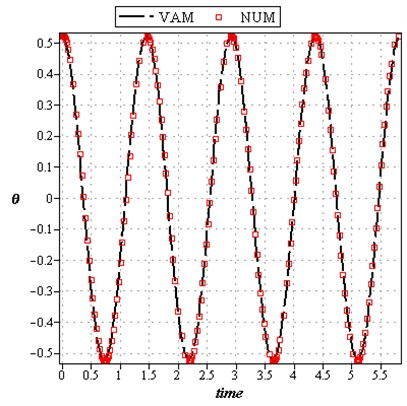a)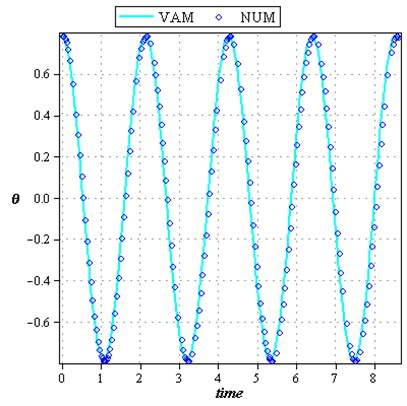b)

Fig. 6Comparison of phase plane of the analytical solution with the numerical solution for: a) L=0.5 m, ω0=1 rad/sec, Y=0.25 m, g=9.81 m/s2, A=π/6, b) L=1 m, ω0=1 rad/sec, Y=0.5 m, g=9.81 m/s2, A=π/4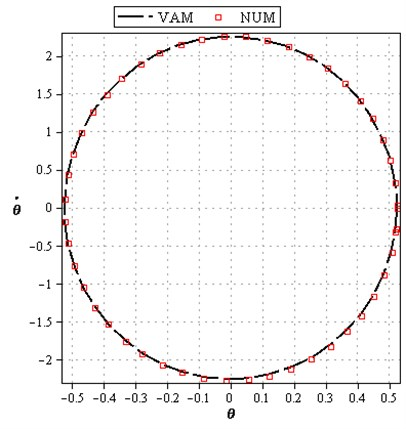a)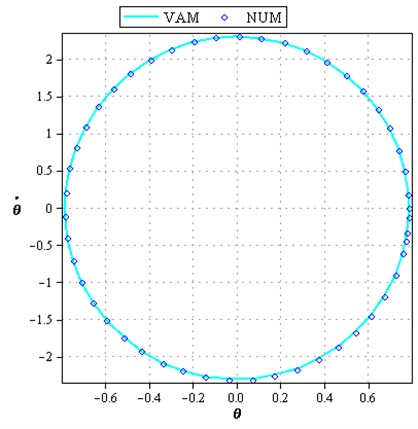b)

## 5. Results and discussions

Some diagrams are illustrated to show the accuracy of the variational approach method with the numerical solution using Runge-Kutta method. Figure 3 represents a comparison of analytical solution of $\theta \left(t\right)$ based on time with the numerical solution for two cases (a): $L=0.5\mathrm{}\mathrm{m}$, $m=20\mathrm{k}\mathrm{g}$, $k=800\mathrm{}\mathrm{N}/\mathrm{m}$, ${F}_{0}=1\mathrm{N}$, ${\omega }_{0}=2\mathrm{}\mathrm{r}\mathrm{a}\mathrm{d}/\mathrm{s}\mathrm{e}\mathrm{c}$, $A=\pi /\text{6}$, (b): $L=1\mathrm{}\mathrm{m}$, $m=10\mathrm{}\mathrm{k}\mathrm{g}$, $k=1200\mathrm{}\mathrm{N}/\mathrm{m}$, ${F}_{0}=1\mathrm{N}$, ${\omega }_{0}=2\mathrm{}\mathrm{r}\mathrm{a}\mathrm{d}/\mathrm{s}\mathrm{e}\mathrm{c}$, $A=\pi /\text{3}$. Figure 4 shows the comparisons of analytical solution of $\stackrel{˙}{\theta }$ based on $\theta \left(t\right)$ with the numerical solution again for two important cases (i) and (ii). In example 2, Figure 5 represents the comparison of analytical solution of $\theta \text{\hspace{0.17em}}\left(t\right)$ based on time with the numerical solution for two cases (a) and (b). It shows that the behavior of the oscillation is periodic. And Figure 6 represents the comparison of $\stackrel{˙}{\theta }$ versus $\theta \text{\hspace{0.17em}}\left(t\right)$ of the analytical solution with the numerical solution for different parameters in two cases to show the accuracy of the method. It has been indicated that VAM has excellent agreement with the numerical solution. It is a simple method and easy to apply to any kind of nonlinear vibration problems.

## 6. Conclusions

In this study a new mathematical tool has been successfully used to study nonlinear vibration equations. We considered two highly nonlinear problems in this paper, the results obtained from the VAM and Runge-Kutta method have an excellent agreement. It has been shown that the obtained solutions are valid for the whole domain. In VAM just one iteration leads us to a high accuracy of the solution. It has been shown that the VAM can be easily extended to any kind of nonlinear vibration equations.